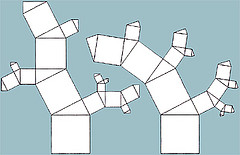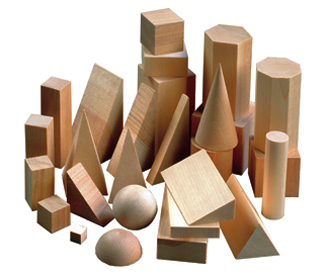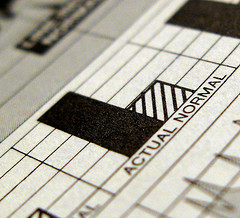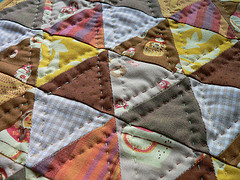FAIL (the browser should render some flash content, not this).Math 8 Honours Ch 1

•  Section 1.1 Basic Operations with integers
•  Section 1.2 Perfect Squares and Cubes
•  Section 1.3 Multiplication Strategies
•  Section 1.4 Order of Operations
•  Section 1.5 Prime Factors, GCF, LCM
•  Section 1.6 Divisibility Rules
•  Section 1.7 Prime Factorization
•  Section 1.8: How to Multiply Like a Master
•  Ch 1 Review: Basic Operations With Integers, Factors, and Divisibility

PPT

•  1.1

•  1.2

•  1.3

•  1.4

•  1.5

•  1.6

•  1.7

Integers and FractionsMath 8 Honours Ch 2

•  Pre Lesson: Basic Operations with Fractions
•  Section 2.1 Solving Equations with Simple Equations
•  Section 2.2 Solving Equations with Fractions and Distributive Properties
•  Section 2.3 Solving Problems with Equations
•  Section 2.4 Solving Equations Involving Reciprocals and Square Roots
•  Section 2.5 Multiplying and Dividing Algebraic Terms
•  Section 2.6 Multiplying and Expanding Polynomials
•  Section 2.7 Factorign GCF and Trinomials
•  Section 2.8 Factoring Difference of Squares
•  Section 2.9 Factoring and Solving Trinomials
•  Pre Lesson: Algebraic Expressions and Combining Like Terms

PPT

•  2.1

•  2.2

•  2.3

•  2.4

•  2.5

•  2.6

•  2.7

Algebra and Factoring

•  Pre Lesson: Basic Operations with Fractions
•  Section 2.1 Solving Equations with Simple Equations
•  Applet for Solving Equations
•  Click for HomeworkMath 8 Honours Ch 3

PPT

•  3.1

•  3.2

•  3.3

•  3.4

•  3.5

•  3.6

•  3.7

Rates , Ratios, and Percentages

•  Section 3.6: Rates and Ratios
•  Section 3.6: Rates and Ratios
•  Section 3.6: Rates and Ratios

•  Click for HomeworkMath 8 Honours Ch 4

•  Supplementary Lesson: What is hte Pythagorean Theorem
•  Section 4.2 Pythagorean Theorem and Triples
•  Section 4.3 Applications and Problems with the Pythagorean Theorem
•  Chapter 4 Review: The Pythagorean Theorem

PPT

•  4.1

•  4.2

•  4.3

•  4.4

•  4.5

•  4.6

•  4.7

Pythagorean Theorem and Relationships

•  Section 4.4: Side Length of a Square
•  Section 4.5:Math 8 Honours Ch 5

PPT

•  5.1

•  5.2

•  5.3

•  5.4

•  5.5

•  5.6

•  5.7

Areas of 2D ShapesMath 8 Honours Ch 6

PPT

•  6.1

•  6.2

•  6.3

•  6.4

•  6.5

•  6.6

•  6.7

Volume of 3D SolidsMath 8 Honours Ch 7

PPT

•  7.1

•  7.2

•  7.3

•  7.4

•  7.5

•  7.6

•  7.7

Linear RelationshipsMath 8 Honours Ch 8

PPT

•  8.1

•  8.2

•  8.3

•  8.4

•  8.5

•  8.6

•  8.7

Probability and StatisticsMath 8 Honours Ch 9

Tessellations